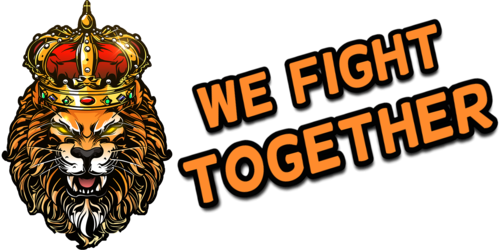search
0# test (from =WFT= Dutch Gaming Clan / Community Voor Squad, Post

januari 1, 1970Uncategorized

=09
=09
=09
=
=09
=09
=09test (from=
=3DWFT=3D Dutch Gaming Clan / Community Voor Squad, Post Scriptum & Ar=
ma 3.)

=09
=09=09
=09=09
=09=09=09
=09=09=09=09=3DWFT=3D Dutch Gamin=
g Clan / Community Voor Squad, Post Scriptum & Arma 3.
=09=09=09
=09=09
=09=09
=09=09=09

The following message has been sent from =3DWFT=3D RobinLollig <[email protected]> (IP: 185.200.13=
2.211) via the contact form at =3DWFT=3D Dutch Gaming Clan / C=
ommunity Voor Squad, Post Scriptum & Arma 3..

test

test1

=09=09=09
=09=09
=
=09=09
=09=09=09
=09=09=09=09

Visit =3DWFT=3D Dutch =
Gaming Clan / Community Voor Squad, Post Scriptum & Arma 3.

=

=09=09=09=09
=09=09=09
=09=09
=09=09
=09